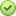# Export multiple tables in GDB to Excel with a new sheet for each table

2665
10
05-16-2018 09:05 AMOccasional Contributor II

Researching to see if there is a way to use Python to export multiple tables in a File Geodatabase to a single Excel file. I would like for each sheet name to have the same name as the table.

My current situation is that I ran a Python script to take all the domains a GDB and create a table within the GDB.

The article referenced from:

``def main():  import arcpy, os  gdb = r"Z:\GIS_Projects\zUpdatesBrian\Scripts\ListDomains\ListDomains.gdb"  arcpy.env.overwriteOutput = True   for dom in arcpy.da.ListDomains(gdb):  if dom.domainType == 'CodedValue':  arcpy.DomainToTable_management(in_workspace=gdb,  domain_name=dom.name,  out_table=os.path.join(gdb, dom.name),  code_field="item",  description_field="descrip",  configuration_keyword="")   print " - domain '{0}' of type '{1}' exported to table".format(dom.name, dom.domainType)  else:  print " - domain '{0}' of type '{1}' skipped".format(dom.name, dom.domainType)  if __name__ == '__main__':  main()‍‍‍‍‍‍‍‍‍‍‍‍‍‍‍‍‍‍‍‍``
Tags (2)
10 RepliesbyMVP Esteemed Contributor

I'm in the same boat: I have multiple tables in a fgdb and would like to export them to a workbook as individual worksheets. Just moments ago, I installed the xlsxwriter module as a package in ArcGIS Pro.  That's the easy part.  Now I'm working on figuring it out.  See: Getting Started with XlsxWriter — XlsxWriter Documentation   ( I just create my Hello.xls...)

Hey Dan, Joshua or Curtis- ever work with xlsxwriter?

can't wait to retire....byMVP Esteemed Contributor

yes ... but not tonightbyMVP Esteemed Contributorcan't wait to retire....byMVP Esteemed ContributorbyMVP Esteemed Contributor

Thanks.  I'll take a look in the morning.

can't wait to retire....byMVP Esteemed Contributor

Getting pretty close, but not quite there.  For some reason, I can only get pandas to write one of my tables out to the target excel spreadhheet, and that depends on where I put writer.save() function:

``import pandas as pdimport arcpyfrom arcpy import envfields = ['TaxingEntity', 'ProjectID']targetXL = r'J:\RDA\junk.xls'#arcpy.env.workspace = r'J:\RDA\Temp2.gdb'#tables = arcpy.ListTables()tables = ['Project101','Project102', 'Project103' ]for table in tables:    if table == 'AllTables_5_16':        pass    elif table == 'NeedTaxEntRecords':        pass    elif table == 'BlakesTaxinEntities':        pass    else:        inTable = table        print (table)        tableArray = arcpy.da.TableToNumPyArray(inTable,fields)        print (tableArray)        writer = pd.ExcelWriter(targetXL)        df = pd.DataFrame(data=tableArray)        df.to_excel(targetXL,table)        writer.save()        del df‍‍‍‍‍‍‍‍‍‍‍‍‍‍‍‍‍‍‍‍‍‍‍‍‍‍``

In this example of my code, I'm only working with 3 of the 100+ tables which is why line 8 is commented and line 9 creates a list of table names.  The problem child seems to be line 25 writer.save(): at it's current location inside the for loop, I only get Project101 written to the target, and I get an error:

IndexError: list index out of range

If I comment it out or put it outside the for loop, I don't get any errors, but I only get Project 103 to get written to the target.

Perhaps a second (or third) set of eyes might see what the issue could be?

can't wait to retire....byMVP Esteemed Contributor

My guess is this line is messing it up:

``df.to_excel(targetXL,table)‍``

Instead of passing the XLS file path, try passing the writer itself.byMVP Esteemed Contributor

Following pandas.DataFrame.to_excel — pandas 0.23.0 documentation , it shows:

``>>> writer = pd.ExcelWriter('output.xlsx')>>> df1.to_excel(writer,'Sheet1')>>> df2.to_excel(writer,'Sheet2')>>> writer.save()‍‍‍‍``

However, when I use 'writer' as shown in lines 1 & 2 (line 24 in my script) the target excel work book does get created at all. However, when I use targetXL , it does get created,with only one sheet.  Weird....

When I take the process out of the loop, and only work with 1 table it works as you and the documentation suggests:

``import pandas as pdimport arcpyfrom arcpy import envfields = ['TaxingEntity', 'ProjectID']targetXL = r'J:\RDA\junk.xls'#arcpy.env.workspace = r'J:\RDA\Temp2.gdb'table = 'Project101'    # just one, not a list now....inTable = tabletableArray = arcpy.da.TableToNumPyArray(inTable,fields)writer = pd.ExcelWriter(targetXL)df = pd.DataFrame(tableArray)df.to_excel(writer,table)writer.save()‍‍‍‍‍‍‍‍‍‍‍‍‍‍‍‍``

When I put print() functions in along the way, I can see each array, and each subsequent dataframe while in the original loop; again weird.  I'll keep hacking.....

can't wait to retire....byMVP Esteemed Contributor

Ha.... Got it....  Notice below where I place the writer variable: outside the for loop.  What was happening is the target xls would get re-initialized each time through the loop.  Now that I set it up outside the loop, it runs like a champ!  Fun stuff!  And I'm getting paid for it!

``import pandas as pdimport arcpyfrom arcpy import envfields = ['TaxingEntity', 'ProjectID']targetXL = r'J:\RDA\ToBlake.xls' ##### <- set up the target outside the loop#arcpy.env.workspace = r'J:\RDA\Temp2.gdb'tables = arcpy.ListTables()writer = pd.ExcelWriter(targetXL)for table in tables:    if table == 'AllTables_5_16':        pass    elif table == 'NeedTaxEntRecords':        pass    elif table == 'BlakesTaxinEntities':        pass    else:        inTable = table        tableArray = arcpy.da.TableToNumPyArray(inTable,fields)        df = pd.DataFrame(tableArray)        df.to_excel(writer,table)        del tableArray        del df        writer.save()‍‍‍‍‍‍‍‍‍‍‍‍‍‍‍‍‍‍‍‍‍‍‍‍``

Sorry to hi-jack your thread Brian M‌, but here you go!

can't wait to retire....